Courses

# Two Hinged Arch (Part - 1) Civil Engineering (CE) Notes | EduRev

## Civil Engineering (CE) : Two Hinged Arch (Part - 1) Civil Engineering (CE) Notes | EduRev

The document Two Hinged Arch (Part - 1) Civil Engineering (CE) Notes | EduRev is a part of the Civil Engineering (CE) Course Structural Analysis.
All you need of Civil Engineering (CE) at this link: Civil Engineering (CE)

Instructional Objectives:

After reading this chapter the student will be able to

1. Compute horizontal reaction in two-hinged arch by the method of least work.
2. Write strain energy stored in two-hinged arch during deformation.
4. Compute reactions developed in two hinged arch due to temperature loading.

Introduction

Mainly three types of arches are used in practice: three-hinged, two-hinged and hingeless arches. In the early part of the nineteenth century, three-hinged arches were commonly used for the long span structures as the analysis of such arches could be done with confidence. However, with the development in structural analysis, for long span structures starting from late nineteenth century engineers adopted two-hinged and hingeless arches. Two-hinged arch is the statically indeterminate structure to degree one. Usually, the horizontal reaction is treated as the redundant and is evaluated by the method of least work. In this lesson, the analysis of two-hinged arches is discussed and few problems are solved to illustrate the procedure for calculating the internal forces.

Analysis of two-hinged arch

A typical two-hinged arch is shown in Fig. 33.1a. In the case of two-hinged arch, we have four unknown reactions, but there are only three equations of equilibrium available. Hence, the degree of statical indeterminacy is one for two hinged arch.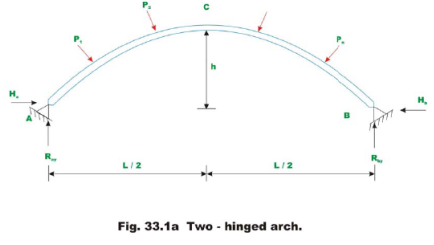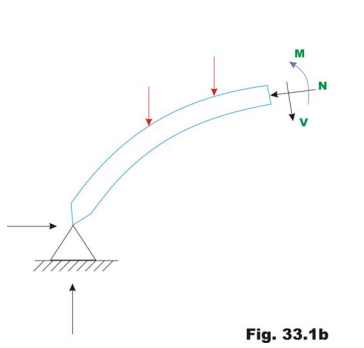The fourth equation is written considering deformation of the arch. The unknown redundant reaction His calculated by noting that the horizontal displacement of hinge B is zero. In general the horizontal reaction in the two hinged arch is evaluated by straightforward application of the theorem of least work (see module 1, lesson 4), which states that the partial derivative of the strain energy of a statically indeterminate structure with respect to statically indeterminate action should vanish. Hence to obtain, horizontal reaction, one must develop an expression for strain energy. Typically, any section of the arch (vide Fig 33.1b) is subjected to shear force V , bending moment M and the axial compression N. The strain energy due to bending Ub is calculated from the following expression.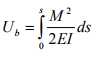(33.1)

The above expression is similar to the one used in the case of straight beams. However, in this case, the integration needs to be evaluated along the curved arch length. In the above equation, s is the length of the centerline of the arch, I is the moment of inertia of the arch cross section, E is the Young’s modulus of the arch material. The strain energy due to shear is small as compared to the strain energy due to bending and is usually neglected in the analysis. In the case of flat arches, the strain energy due to axial compression can be appreciable and is given by,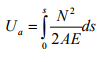(33.2)

The total strain energy of the arch is given by,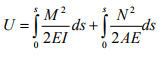(33.3)

Now, according to the principle of least work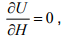where H is chosen as the redundant reaction.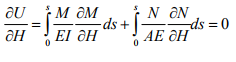(33.4)

Solving equation 33.4, the horizontal reaction H is evaluated.

Symmetrical two hinged arch

Consider a symmetrical two-hinged arch as shown in Fig 33.2a. Let C at crown be the origin of co-ordinate axes. Now, replace hinge at B with a roller support. Then we get a simply supported curved beam as shown in Fig 33.2b. Since the curved beam is free to move horizontally, it will do so as shown by dotted lines in Fig 33.2b. Let M0 and N0 be be the bending moment and axial force at any cross section of the simply supported curved beam. Since, in the original arch structure, there is no horizontal displacement, now apply a horizontal force H as as shown in Fig. 33.2c. The horizontal force H should be of such magnitude, that the displacement at B must vanish.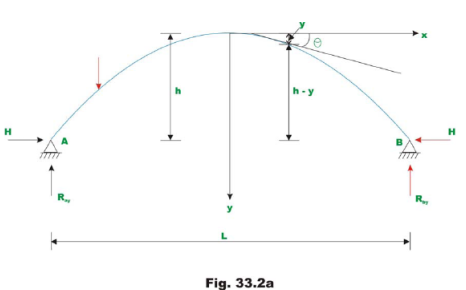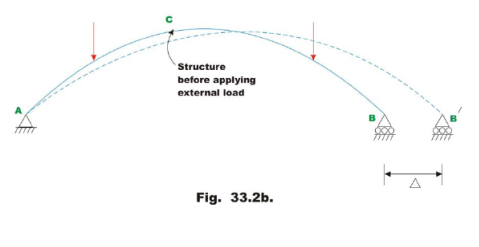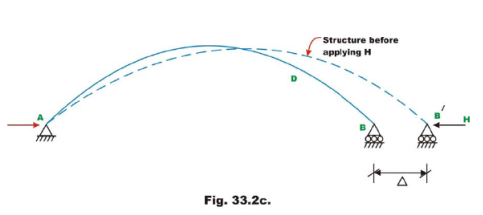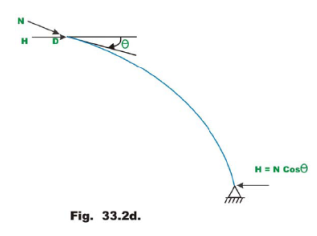From Fig. 33.2b and Fig 33.2c, the bending moment at any cross section of the arch (say D ), may be written as

M = M0 - H(h - y)                                (33.5)

The axial compressive force at any cross section (say D) may be written as

N = N0 + H cos θ                                  (33.6)

Where θ is the angle made by the tangent at D with horizontal (vide Fig 33.2d). Substituting the value of M and N in the equation (33.4),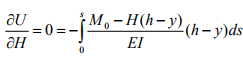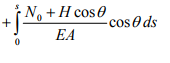(33.7a)

Let,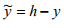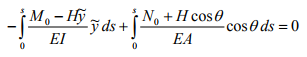(33.7b)

Solving for H , yields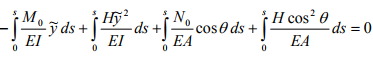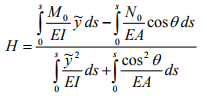(33.8)

Using the above equation, the horizontal reaction H for any two-hinged symmetrical arch may be calculated. The above equation is valid for any general type of loading. Usually the above equation is further simplified. The second term in the numerator is small compared with the first terms and is neglected in the analysis. Only in case of very accurate analysis second term s considered. Also for flat arched, θ ≅1cos as θ is small. The equation (33.8) is now written as,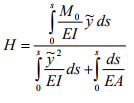(33.9)

As axial rigidity is very high, the second term in the denominator may also be neglected. Finally the horizontal reaction is calculated by the equation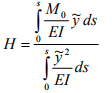(33.10)

For an arch with uniform cross section EI is constant and hence,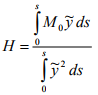(33.11)

In the above equation, M0 is the bending moment at any cross section of the arch when one of the hinges is replaced by a roller support.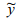is the height of the arch as shown in the figure. If the moment of inertia of the arch rib is not constant, then equation (33.10) must be used to calculate the horizontal reaction H .

Offer running on EduRev: Apply code STAYHOME200 to get INR 200 off on our premium plan EduRev Infinity!

## Structural Analysis

30 videos|122 docs|28 tests

,

,

,

,

,

,

,

,

,

,

,

,

,

,

,

,

,

,

,

,

,

;